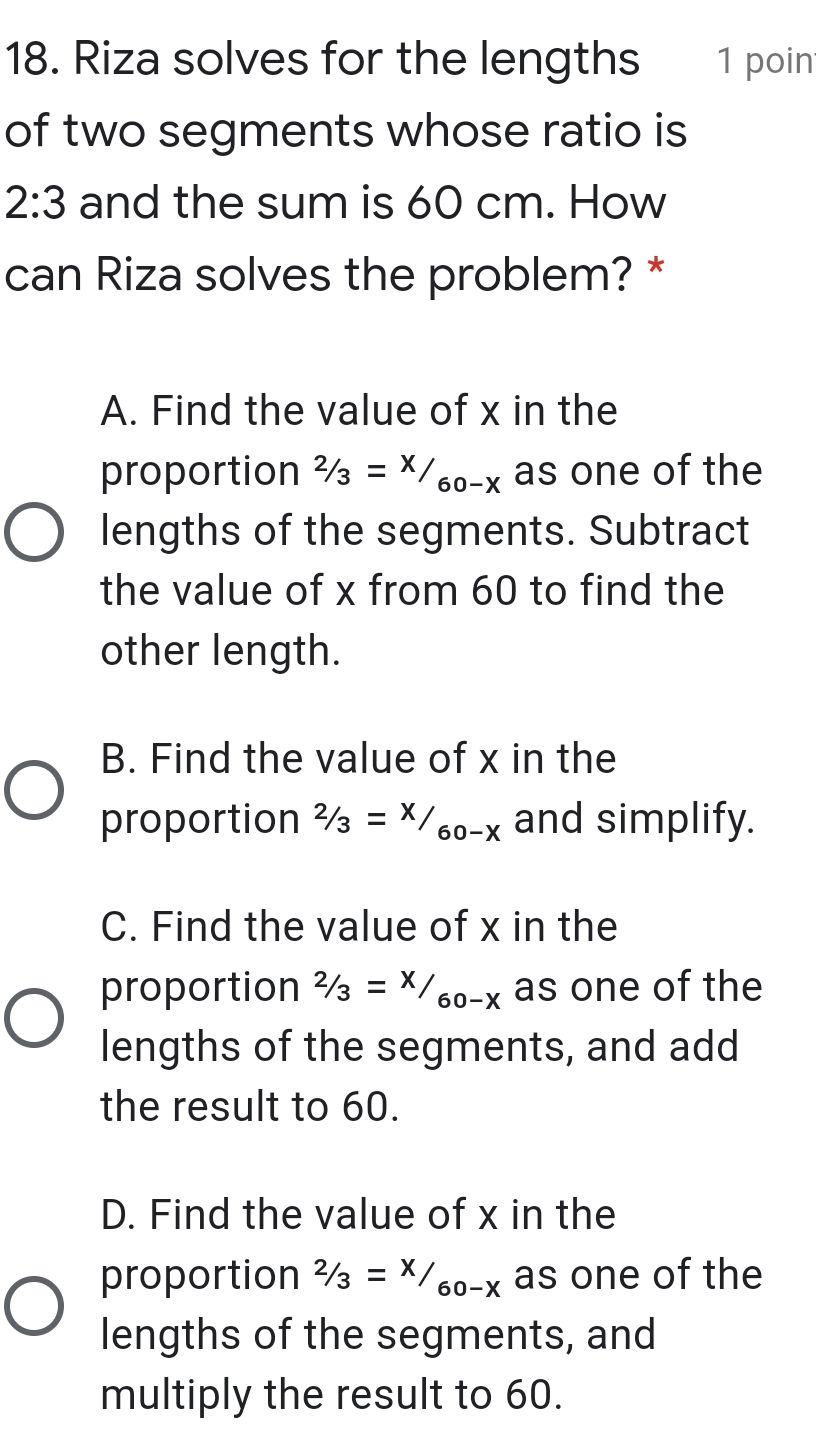### Still have math questions?

Algebra
Question18. Riza solves for the lengths of two segments whose ratio is

$$2 : 3$$ and the sum is $$60 cm$$ . How can Riza solves the problem? * A. Find the value of $$x$$ in the proportion $$2 / 3 = x / 60 - x$$ as one of the lengths of the segments. Subtract the value of $$x$$ from $$60$$ to find the other length. B. Find the value of $$x$$ in the proportion $$2 / 3 = x / 60 - x$$ and simplify. C. Find the value of $$x$$ in the proportion $$2 / 3 = x / 60 - x$$ as one of the lengths of the segments, and add the result to $$60$$ . D. Find the value of $$x$$ in the proportion $$2 / 3 = x / 60 - x$$ as one of the lengths of the segments, and multiply the result to $$60$$ .